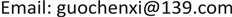﻿ 关于物质概念的思考 Thinking about the Concept of Matter

Modern Physics
Vol.08 No.04(2018), Article ID:25731,8 pages
10.12677/MP.2018.84019

Thinking about the Concept of Matter

Chenxi Guo

China Academy of Railway Science, BeijingReceived: Jun. 11th, 2018; accepted: Jun. 26th, 2018; published: Jul. 3rd, 2018ABSTRACT

In this paper, the concept of matter is examined in plain nature-views; and it emphasizes the identity of mass and energy; the intrinsic property of matter is electromagnetics; and the law of conservation of energy and the principle of constancy of light velocity are the common rule in the evolution process of the material world. From the above understanding of matter, it reconsiders the laws of Newton’s classical mechanics, and hereby consolidates the fundamental relations between energy, force, momentum, inertial mass and gravitational mass in mechanics. The application scope of modified Newton’s mechanics law is extended to the relativistic domain, giving an example to calculate the critical radius of a black hole of one solar mass.

Keywords:Matter, Inertia, Energy, Mass, Relativity1. 引言

2. 能量守恒

“实体在现象的一切变化中持存着，它的量在自然中既不增加也不减少”。“产生和消失不是那产生或消失的东西的变化。变化是一种实存的方式，它紧跟着同一个对象的另一种实存方式之后。因此一切变化之物都是保留着的，只是它的状态变更了  ”。没有和外界交换时，物质(能量E)不能增多，也不会减少，即物质不能随时间而变化，物质是永恒的，即能量守恒定律：

$\frac{\text{d}E}{\text{d}t}=0$ (1)

n个系统之间存在能量交换时，有：

$\sum \frac{\text{d}{E}_{i}}{\text{d}t}=0$ (2)

3. 物质的电磁属性

4. 物体的惯性

$E=\gamma {m}_{0}{c}^{2}$ (3)

$\gamma =\frac{1}{\mathrm{cos}\theta }=\frac{1}{\sqrt{1-{\left(\frac{v}{c}\right)}^{2}}}$

${l}^{\prime }=l\mathrm{cos}\theta =l\sqrt{1-{\left(\frac{v}{c}\right)}^{2}}$Figure 1. In velocity space of a moving object in speed v relative to S

5. 力的概念

$F=\frac{\text{d}E}{\text{d}s}$ (4)

6. 基本力学定律的物理意义

6.1. 惯性定律

$\frac{\text{d}E}{\text{d}s}=\frac{\text{d}E}{v\text{d}t}=0$ (5)

6.2. 牛顿第2定律

$\frac{\text{d}E}{\text{d}s}=\frac{\text{d}}{\text{d}s}\left(\frac{{m}_{0}{c}^{2}}{\sqrt{1-{\frac{v}{{c}^{2}}}^{2}}}\right)=\frac{\text{d}}{v\text{d}t}\left(\frac{{m}_{0}{c}^{2}}{\sqrt{1-{\frac{v}{{c}^{2}}}^{2}}}\right)$

$F=\frac{\text{d}E}{\text{d}s}={m}_{0}{\left(1-\frac{{v}^{2}}{{c}^{2}}\right)}^{-\frac{3}{2}}\frac{\text{d}v}{\text{d}t}={m}_{0}{\left(1-\frac{{v}^{2}}{{c}^{2}}\right)}^{-\frac{3}{2}}a$

$F={m}_{0}{\gamma }^{3}a$ (6)

$m={m}_{0}{\left(1-\frac{{v}^{2}}{{c}^{2}}\right)}^{-\frac{3}{2}}$

$v\ll {c}^{2}$ 时，得出牛顿第2定律的经典形式：

$F={m}_{0}a$ (7)

6.3. 牛顿第3定律

$\left(\frac{\text{d}{E}_{1}}{\text{d}s}+\frac{\text{d}{E}_{2}}{\text{d}s}\right)=0$$\left(\frac{\text{d}{E}_{1}}{\text{d}s}+\frac{\text{d}{E}_{2}}{\text{d}s}\right)=0$

${F}_{1}=-{F}_{2}$ (8)

$F={m}_{0}{\gamma }^{3}a={m}_{0}{\gamma }^{3}\left({a}_{x}i+{a}_{y}j\right)={m}_{0}{\gamma }^{3}{a}_{x}i+{m}_{0}{\gamma }^{3}{a}_{y}j$

${F}_{x}={m}_{0}{\gamma }^{3}{a}_{x}$${F}_{y}={m}_{0}{\gamma }^{3}{a}_{y}$

7. 动量

$\frac{\text{d}p}{\text{d}t}=F$ (9)

$p=\int F\text{d}t=\int {\gamma }^{3}{m}_{0}\frac{\text{d}v}{\text{d}t}\text{d}t=\int {\gamma }^{3}{m}_{0}\text{d}v=\gamma {m}_{0}v+{P}_{0}$

$p\left(v=0\right)=0$ ，则：

$p=\gamma {m}_{0}v=E\frac{v}{{c}^{2}}$ (10)

$p=E\frac{v}{{c}^{2}}=\frac{E}{c}=\frac{h}{\lambda }$

${E}^{2}-{\left(pc\right)}^{2}={\left({m}_{0}{c}^{2}\right)}^{2}$ (11)

8. 物质的引力属性——引力定律的能量表述

$F=G\frac{{m}_{1}{m}_{2}}{{r}^{2}}=G\frac{{E}_{1}{E}_{2}}{{c}^{4}{r}^{2}}=g\frac{{E}_{1}{E}_{2}}{{r}^{2}}$

$\Delta E={\int }_{{r}_{0}}^{\infty }g\frac{{M}_{sun}{c}^{2}E}{{r}^{2}}\text{d}r$

$\begin{array}{l}\Delta E={\int }_{{r}_{0}}^{\infty }g\frac{{M}_{sun}{c}^{2}{E}_{0}}{{r}^{2}}\text{d}r=g\frac{{M}_{sun}{c}^{2}{E}_{0}}{{r}_{0}}=G\frac{{M}_{sun}{E}_{0}}{{r}_{0}{c}^{2}}\\ \frac{\Delta E}{{E}_{0}}=\frac{G{M}_{sun}}{{r}_{0}{c}^{2}}\approx 2.122×{10}^{-6}\end{array}$

$\Delta E={E}_{0}$ 时，光子能量损失殆尽，将不能摆脱引力的束缚，这时可以得到星体的最大临界半径 ${r}_{c}$ 应满足：

$\frac{\Delta E}{{E}_{0}}=\frac{GM}{{r}_{\text{c}}{c}^{2}}=1$${r}_{c}=\frac{GM}{{c}^{2}}$

${r}_{c}=\frac{GM}{{c}^{2}}=1477\text{\hspace{0.17em}}\text{m}$

9. 结论

Thinking about the Concept of Matter[J]. 现代物理, 2018, 08(04): 154-161. https://doi.org/10.12677/MP.2018.84019

1. 1. 爱因斯坦, 著. 狭义与广义相对论浅说[M]. 杨润殷, 译. 北京: 北京大学出版社, 2006: 36.

2. 2. 康德, 著. 纯粹理性批判[M]. 邓晓芒, 译. 北京: 人民出版社, 2004: 170-174.

3. 3. 爱因斯坦, 著. 狭义与广义相对论浅说[M]. 杨润殷, 译. 北京: 北京大学出版社, 2006: 177.

4. 4. 罗素著, 何兆武, 李约瑟. 西方哲学史[M]. 上卷. 北京: 商务印书馆, 2005: 52.

5. 5. 罗素著, 何兆武, 李约瑟. 西方哲学史[M]. 上卷. 北京: 商务印书馆, 2005: 74.